### IMO Shortlist 2014 problem G1

Kvaliteta:
Avg: 3,0
Težina:
Avg: 6,5

The points$P$ and$Q$ are chosen on the side$BC$ of an acute-angled triangle$ABC$ so that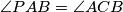$\angle PAB = \angle ACB$ and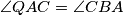$\angle QAC = \angle CBA$. The points$M$ and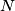$N$ are taken on the rays$AP$ and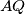$AQ$, respectively, so that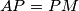$AP = PM$ and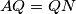$AQ = QN$. Prove that the lines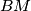$BM$ and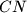$CN$ intersect on the circumcircle of the triangle$ABC$.

(Georgia)

Izvor: https://www.imo-official.org/problems/IMO2014SL.pdf